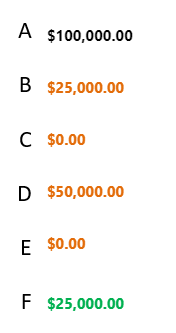# Two fields do a calculation

Can you make two field do math dynamically in the form? for example, quality is 5 and the unit price is 5.00
the total would be 25.00

Hello @jktodd007,

Yes, you can add the on change event on the Price field and do any calculation. Please see the code sample below.

``````fd.spRendered(function() {
fd.field('price').\$on('change', function() {

var el1 = parseInt(fd.field('quantity').value);
var el2 = parseInt(fd.field('price').value);

fd.field('Total').value = el1 * el2;
});
});``````

Is there a way to do this with the below data?that even if B or C or D or E is left blank it will still calculate with F.

The formula of the calculation is F = A - (B+C+D+E)

Hello @Jamail_Serio,

Yes, you can use the same approach. Please see teh code sample below.

``````function calculation() {
var fieldA = 0;
var fieldB = 0;
var fieldC = 0;

if(fd.field('FieldA').value) {
var fieldA = fd.field('FieldA').value;
}

if(fd.field('FieldB').value) {
var fieldB = fd.field('FieldB').value;
}
if(fd.field('FieldC').value) {
var fieldC = fd.field('FieldC').value;
}

fd.field('FieldF').value = fieldA - (fieldB + fieldC);
}

calculation();

fd.field('FieldA').\$on('change', calculation);
fd.field('FieldB').\$on('change', calculation);
fd.field('FieldC').\$on('change', calculation);
``````

Please use fields' internal names in the code.

1 Like